Incredibly Helpful GMAT Tutor NYC & Online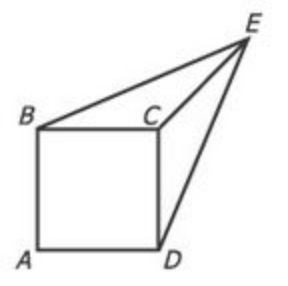# In the figure above, each side of square ABCD has length 1, the length of line segment CE is 1, and the length of line segment BE is equal to the length of line segment DE. What is the area of the triangular region BCE?

(A) 1/3
(B) √2/4
(C) 1/2
(D) √2/2
(E) 3/4

This is a tough geometry question from the GMAT prep tests (I think it's from exam pack 1, tests 3/4). The first time I saw this one I made the mistake of assuming a 3 dimensional figure. The question is much easier once you put the shape in 2 dimensions dropping point E onto the diagonal of square ABCD. At that point it becomes a set of somewhat straightforward inferences. Again, this is a very difficult question and a great majority of people won't get it correct. So don't worry if you get a little lost. What's key is seeing that the solution is basic. There's isn't any difficult math involved. The tough part is figuring out what the question is telling you.

## What is the area of the triangular region BCE? Let's figure it out!

There are two important inferences to make:

1. For a square always define the diagonal. It comes up a ton on GMAT geometry questions.
2. If you're looking for the area of a triangle you'll need a height. If not dealing with a right triangle go ahead and always draw an altitude/height.

Once you've done those two things finding the area of the triangular region BCE is a piece of cake.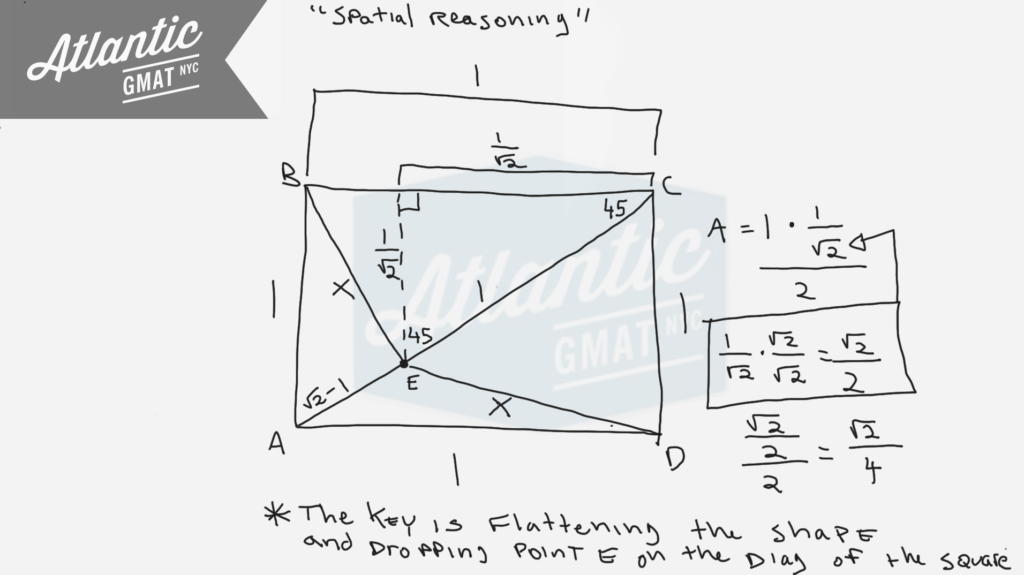## More challenging GMAT geometry questions with right isosceles triangles

Here is another challenging geometry question with 45-45-90 triangles: The perimeter of a certain isosceles right triangle is 16 + 16√2. What is the length of the hypotenuse of the triangle?

A small, rectangular park has a perimeter of 560 feet and a diagonal measurement of 200 feet. What is its area, in square feet?

(A) 19,200
(B) 19,600
(C) 20,000
(D) 20,400
(E) 20,800

This is a very basic GMAT geometry question: area of a rectangle given the perimeter and diagonal. System of equations. Again, the setup is easy peasy. It gets a bit tricky once you need to simplify to an answer. Depending on how you approach the execution you might get stuck. In addition, the answer choices are bunched closely together so you can't really estimate/approximate.

What should you do if encountered with this type of situation on your GMAT? Panic! Just kidding. Remind yourself that there's likely a simple way to approach things. Remember that unless a number is prime you can pull it apart. Often then there's an easier "shape" to a number depending on what you're trying to do.

In this case, by pulling out some factors we can:

1. Do easier math with known multiples
2. Divide by two

Also, with exponents often you end up factoring. So given that you have a bunch of exponents present factoring should come to mind. These simple setup/tricky execution questions aren't uncommon so it's important to work on being flexible in how you approach your GMAT arithmetic. Hope this is helpful. Comment with any questions!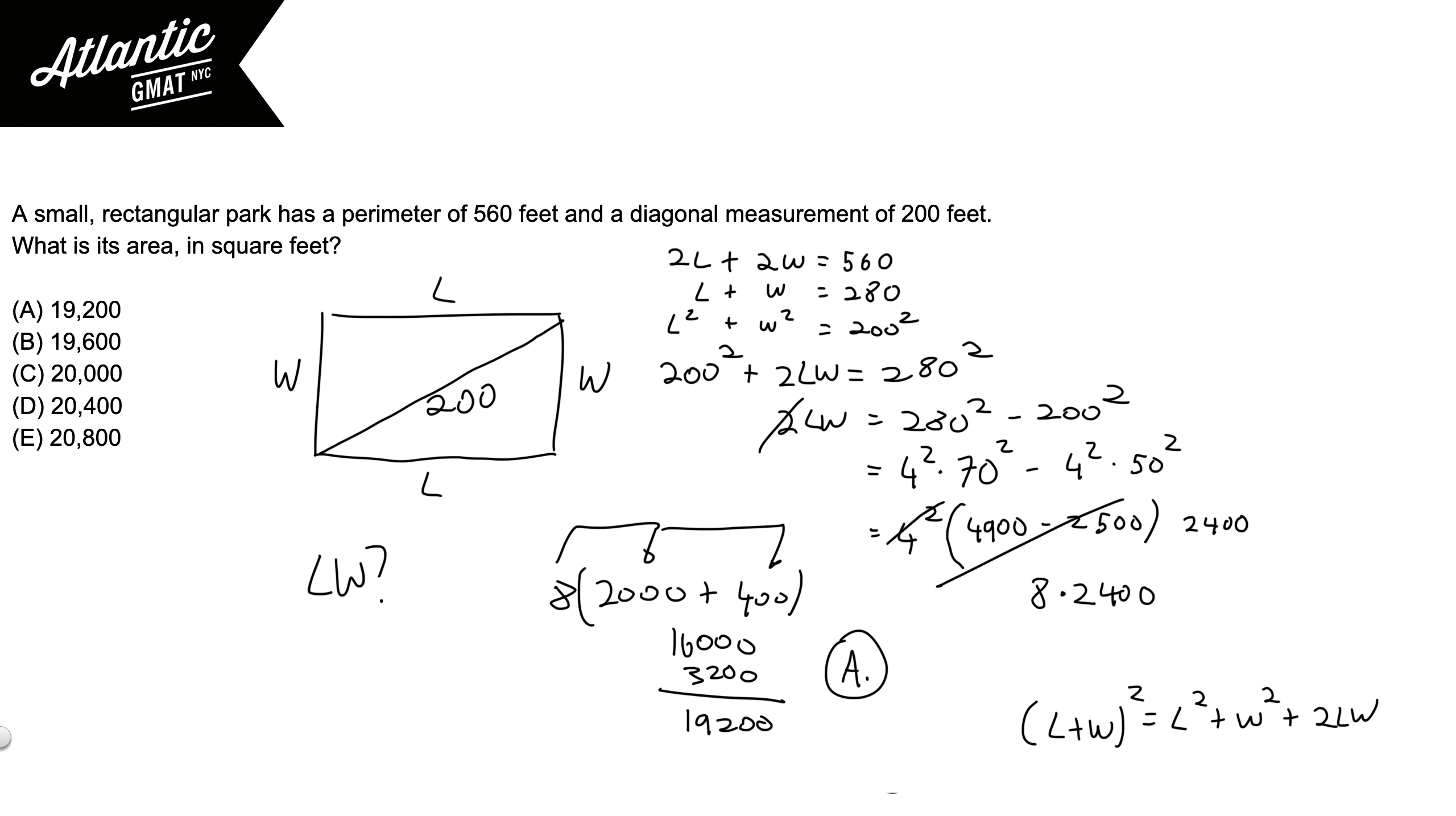## Video Solution: A small, rectangular park has a perimeter of 560 feet and a diagonal measurement of 200 feet. What is its area, in square feet?

In a certain sense these questions are quite different but I think what's challenging about them is the same. They have a bunch of algebra to get to the answer and untangling that is the point at which most people get stuck. The perimeter of a certain isosceles right triangle is 16 + 16√2. What is the length of the hypotenuse of the triangle?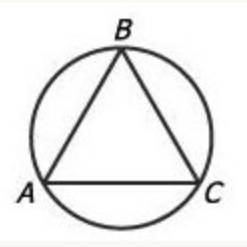In the figure above, equilateral triangle ABC is inscribed in the circle. If the length of arc ABC is 24, what is the approximate diameter of the circle?

(A) 5
(B) 8
(C) 11
(D) 15
(E) 19

It's key to know that an equilateral triangle has equal sides and equal angles measuring 60 degrees each. So each angle of an equilateral triangle inscribed in a circle cuts off 1/3 of the circle. Why is that? Review the basics! An angle on the circumference of a circle cuts off twice that angle's measure. So a 60 degree angle on the edge of a circle cuts off 120 degrees or 1/3 of a circle (120/360 degrees). A 60 degree angle in the center of a circle only cuts off 60 degrees (it doesn't have the extra space to open up). Examples below to illustrate this. Also, there's a special case for triangles that have the diameter of a circle as their base. Because the diameter is a 180 degree central angle, the angle opposite on the circumference of the circle must be 90 degrees. Not relevant for this question but good to know.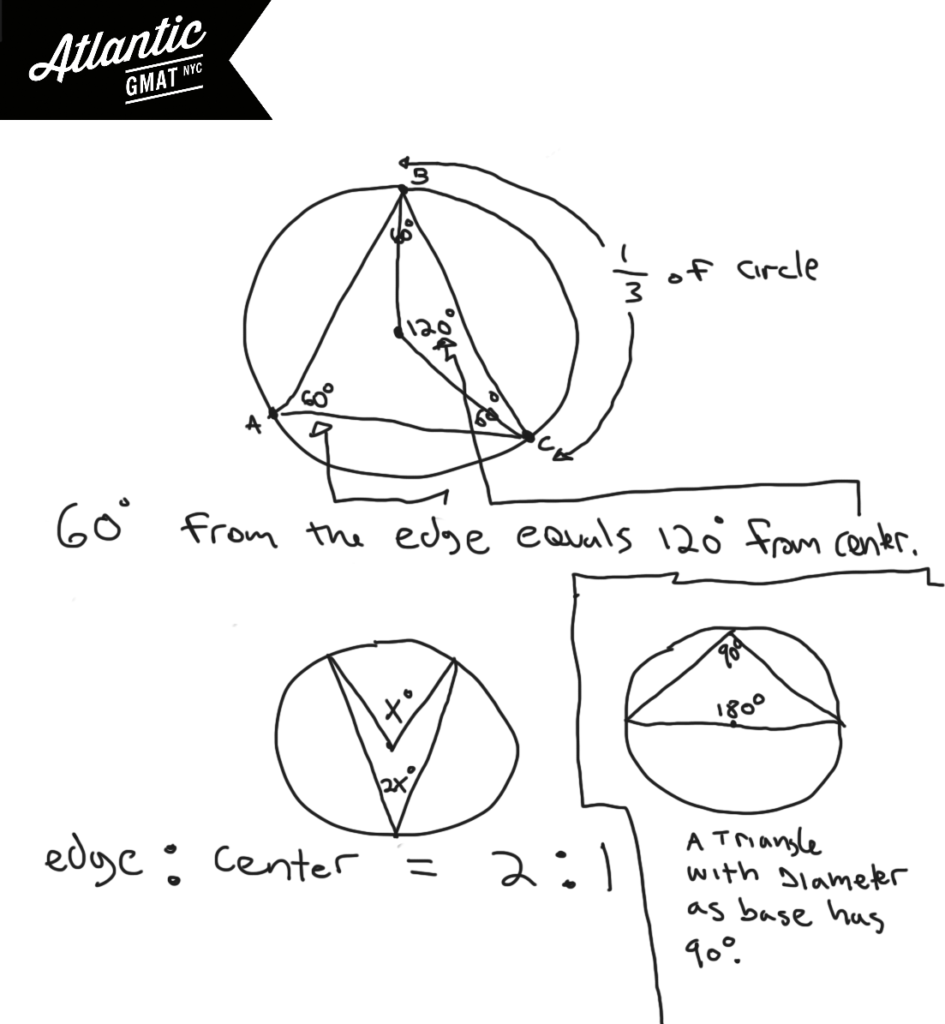How much of the circle is arc ABC? It's covering the same part of the circumference as two of the 60 degree angles from triangle ABA. Each of those angles represent 1/3 of the circle so arc ABC is 2/3 of the circle. If 2/3 is 24, 1/3 of the circle's circumference is 12, and the entire circumference is 36. So Pi*diameter = circumference. Pi*D = 36. D = 36/Pi. D = 36/3.14. A little less than 12. 11 is by far the closest answer choice. A little rusty feeling on these GMAT geometry rules? The rules are the easy part of GMAT studying. Get your fundamentals in shape ASAP. Avoid churning through questions before getting your basics GMAT quant organized. You don't want to waste good material and it's much harder to focus on the basics while working on tougher/trickier GMAT questions. Comment with any questions or additions. Happy studies!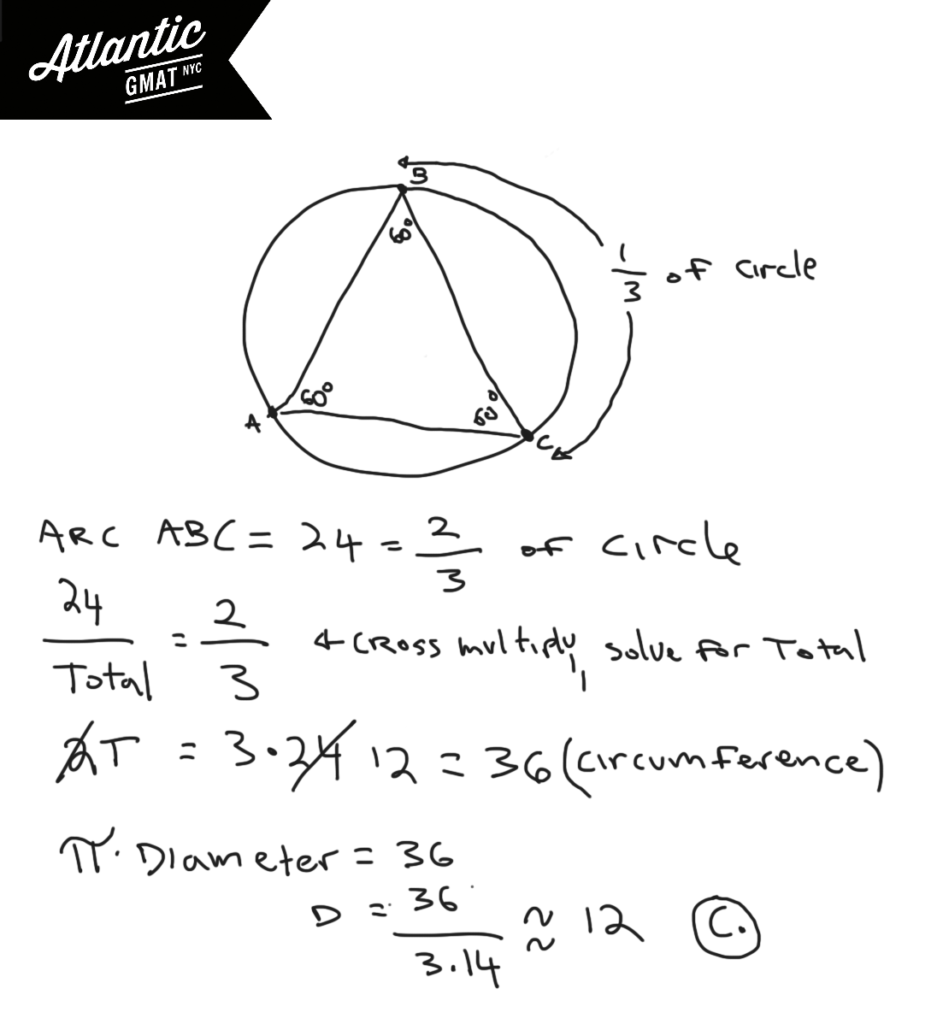A right circular cone is inscribed in a hemisphere so that the base of the cone coincides with the base of the hemisphere. What is the ratio of the height of the cone to the radius of the hemisphere?

(A) √3:1
(B) 1:1
(C) 1/2/1
(D) √2:1
(E) 2:1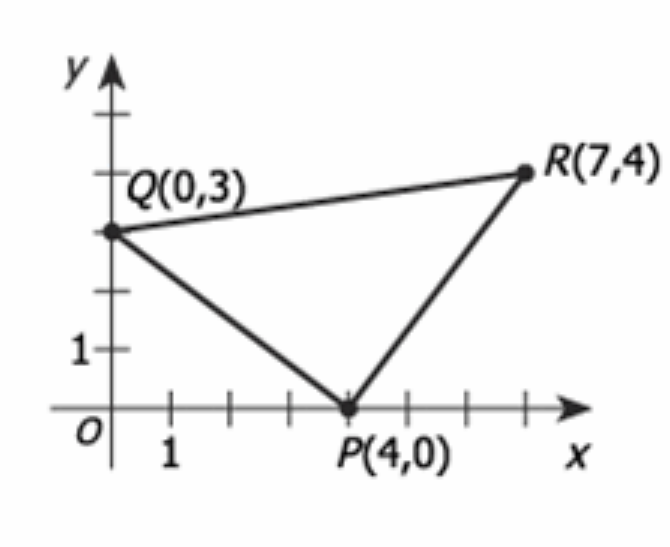In the rectangular coordinate system above, the area of triangular region PQR is

(A) 12.5

(B) 14

(C) 10√2

(D) 16

(E) 25

For GMAT geometry questions be active with diagrams. Don't be satisfied with what you're given. Try to make simple additions that provide more information (inferences). In general when trying to find an area on the coordinate plane you need to make a square, rectangle, or at the least, a right triangle. Right angles make measurement possible. Also, be open to the idea of adding/subtracting the areas of two or more shapes in order to solve for the desired area. In this case I'd draw two extra lines to make a rectangle.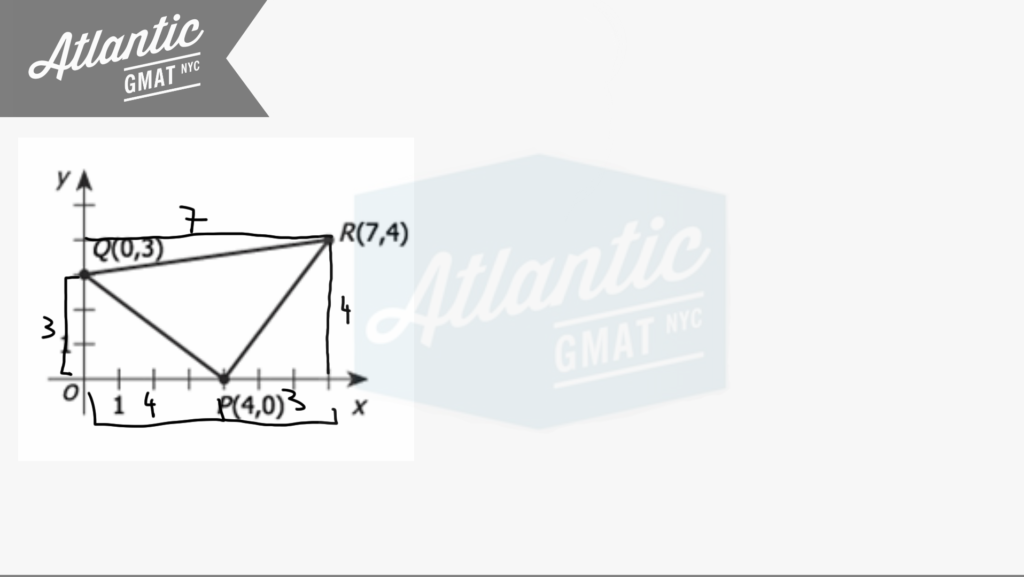With that you should notice that you've created extra triangles and that those are right triangles. On GMAT geometry right triangles are your friends because you can do Pythagorean theorem and calculating are uses on the sides of the triangle (there's no need to drop an altitude). Now you can calculate the area of the rectangle and then subtract from that the areas of the three right triangles. That will leave the area of the middle triangle. Comment with any question or additions. Happy studies!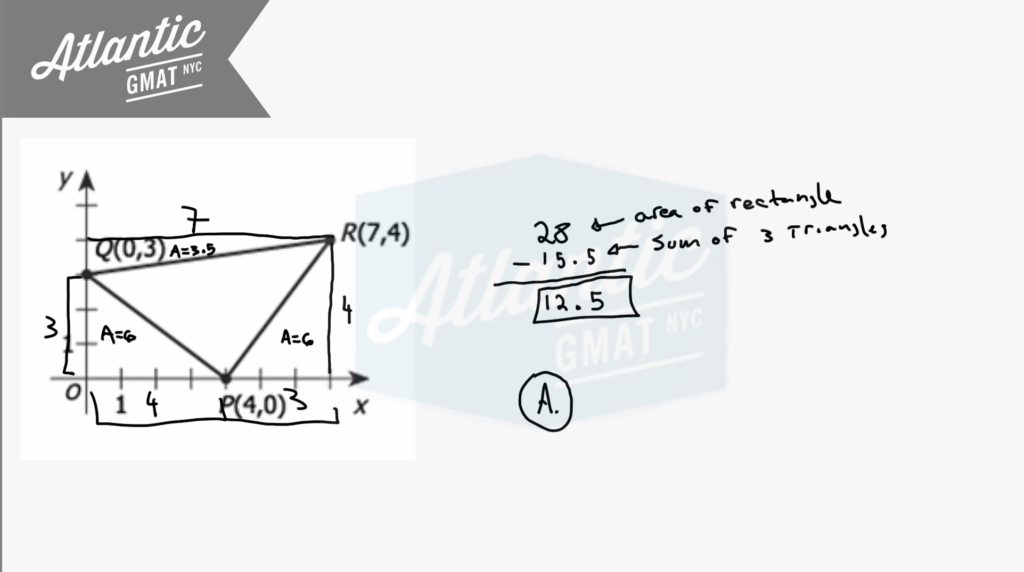To see it done live here is a video solution:

# CONTACT

Atlantic GMAT Tutoring

405 East 51st St.

NY, NY 10022

(347) 669-3545